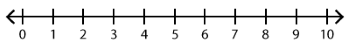##### Notes

Daily Math Practice provides a week's worth of problems ideal for morning work or review. Week 23 reviews standards from previous weeks and supports Common Core Mathematics Standards K.OA.A.1, K.OA.A.2, & K.OA.A.3.

##### Print Instructions

NOTE: Only your test content will print.
To preview this test, click on the File menu and select Print Preview.

See our guide on How To Change Browser Print Settings to customize headers and footers before printing.

# Kindergarten - Daily Math Practice - Week 23 (Kindergarten)

Print Test (Only the test content will print)
 DAILY MATH PRACTICE - WEEK 23 DAY 1 DAY 2 Add. Use the number line.1 + 9 = Subtract. Use the number line.6 - 3 = Kelly gets 5 gifts. She opens 3. How many gifts does Kelly have left to open?Cross off the correct number of pictures:Answer:gifts Sara is feeding her pets. It takes her 4 minutes to feed her dog. It takes 3 minutes to feed her cat. How long does it take Sara to feed her dog and cat?Write an addition sentence:Answer:minutes Circle the addition sentence for the model.7 + 3 = 103 + 7 = 10 Circle the addition sentence for the model.5 + 5 = 106 + 4 = 10 Start at 15 and count forward to 22. Circle the number that comes next.15, 16, 17, 18, 19, 20, 21, 22, 23, 24, 25 Circle the object shaped like a cone.DAILY MATH PRACTICE - WEEK 23 DAY 3 DAY 4 Add. Use the number line.4 + 2 = Subtract. Use the number line.8 - 2 = Jack cooks 5 ears of corn. He eats 1 ear of corn. How many ears of corn are left?Cross off the correct number of pictures:Answer:ears of corn There are 3 students who play flute in the music class. There are 7 students who play trumpet. How many students play flute and trumpet?Write an addition sentence:Answer:students Circle the addition sentence for the model.1 + 9 = 108 + 2 = 10 Circle the addition sentence for the model.3 + 7 = 104 + 6 = 10 Count forward by tens. Circle the number that comes after 80.10, 20, 30, 40, 50, 60, 70, 80, 90 Write the missing number.11 = 1 ten +onesYou need to be a HelpTeaching.com member to access free printables.